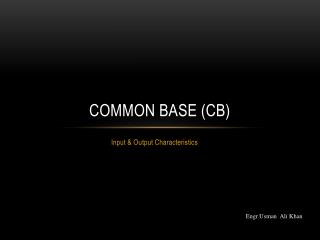Download PresentationCommon Base (CB)

# Common Base (CB) - PowerPoint PPT PresentationDownload Presentation## Common Base (CB)

- - - - - - - - - - - - - - - - - - - - - - - - - - - E N D - - - - - - - - - - - - - - - - - - - - - - - - - - -
##### Presentation Transcript

1. Common Base (CB) Input & Output Characteristics Engr.Usman Ali Khan

2. Contents • CB, CE and CC • CB Circuit • CB Input Characteristics • CB Output Characteristics

3. BJT Configurations • Common Base (CB) • Common Emitter (CE) • Common Collector (CC)

4. Common Base (CB) E C Input Output B Base is common both to input signal and output signal

5. Common Emitter (CE) C B Output Input E Emitter is common both to input signal and output signal

6. Common Collector (CC) C Input B Output E Collector is common both to input signal and output signal

7. CB Voltages and Currents NPN PNP C C IC IC B B IB IB E IE IE E VBE = 0.7 VEB = 0.7 VBE = -VEB

8. CB Input Characteristics IE IC E C Output Side Input Side VBE VBC B To observe the behavior of Base-Emitter junction when VBC is fixed

9. CB Input Characteristics IE VCB = 25V VCB = 10V VCB = 0V 0.7 VBE

10. CB Output Characteristics IE IC E C Output Side Input Side VBE VBC B To observe the behavior of Base-Collector junction when IE is fixed

11. CB Output Characteristics Break down Region or Punch Through Region (Depletion Regions Overlap) IC Active Region IE = 4mA IE = 3mA Saturation Region IE = 2mA Junction Forward Biased IE = 1mA Junction Reverse Biased VBC -1 0 5 10

12. Home Work • Example: 4.2, 4.3 • End Problems: 4.6  4.7

13. Common Emitter (CE) Input & Output Characteristics

14. Contents • β • CE Configuration • CE Input Characteristics • CE Output Characteristics

15. C IC VCB B IB VCE Output Input E VBE IE CE Configuration IE = IC + IB VCE = VCB + VBE VCE = VCB + 0.7 Input Current = IB Input Voltage = VBE Output Current = IC Output Voltage = VCE

16. IC = 1mA IB = 10 µA β =?

17. CE Input Characteristics IB VCE = 10V VCE = 20V 0.7 VBE

18. CE Output Characteristics Break down Region or Punch Through Region (Depletion Regions Overlap) IC Active Region IB = 40 µ A IB = 30 µ A Saturation Region IB = 20 µ A IB = 10 µA Junction Reverse Biased VCE 5 10 15 20

19. Home Work • CC Characteristics • Example: 4.4  4.6 • End Problems: 4.8  4.17

20. Home task • Make CC(Common Collector) Configuration and draw input output characteristics…